## 20: The Chronometer Escapement

The most important advantage that the Chronometer Escapement has over the Swiss Lever is that lubrication of the escape wheel teeth is not required: the balance's impulse pallet and the escape tooth appear to roll together rather than to slide across one another (as in the Swiss Lever), so there is much less friction in the Chronometer escapement. Since the lubricant may change viscosity as the temperature changes, and may even dry up over time, it is preferable not to lubricate the escapement unless necessary: since the Chronometer escapement has a much lower friction loss, the ability to make it run dry would result in a more consistent timekeeper.

While the principles of this escapement are straightforward, the drawing is difficult to create, and there is plenty of math involved. Draw a 15 tooth escape wheel inside a 6 inch diameter circle.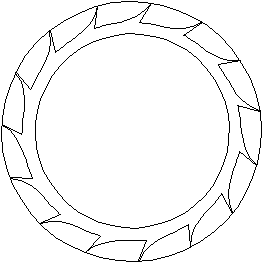Draw a 3.25 inch line from the center of the escape wheel to the edge of the circle. Then draw a vertical line, which will represent the detent, a quarter of an inch away from the edge of the circle. Rotate this line clockwise by 45ş, and place it over the center of the circle and crossing the detent line to create a triangle. The top right corner will become the balance's circle center. To calculate the distance (T) between the two circle centers: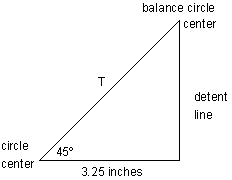let T = 3.25 / cos 45 = 4.596"

You need to find the radius of the impulse pallet's circle (X), that is, the circle that will trace the path of the impulse pallet. If the escape wheel rotates by 22ş (24ş less 2ş for drop) during impulse, you could draw the following triangles:B = 3 cos 11 = 2.945 T = A + B = 4.596 A = 4.596 - 2.945 = 1.651 C = 3 sin 11 = 0.572

X2 = A2 + C2 = 1.6512 + 0.5722

X = 1.747

Draw a circle with a radius of 1.747 inches and place its center on the point where the detent line and the T line meet. Another method could be used to calculate X: it is shown on page 73.

Once the impulse portion of the escapement it finished, it is necessary to calculate the dimensions of the discharging pallet's circle and the gold spring's circle for the detent. If the discharging pallet rotates by 30ş, and the gold spring rotates by 2ş, during discharge, we get another set of triangles. (These are not drawn to scale.)

 R cos 30 + G cos 2 = 6.5 R sin 30 = G sin 2 ...and solve the equations. 0.5 R = 0.035 G so R = 0.07 G Substituting: 0.866 R + 0.999 G = 6.5 (0.866 x 0.07) G + 0.999 G = 6.5 1.060 G = 6.5 G = 6.132" R = 0.07 G = 0.07 x 6.132 R = 0.429"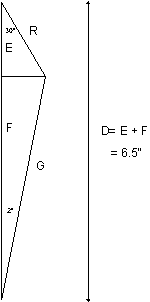Draw a circle with a radius of 0.429" and place it such that its center lies on the point where the detent line and line T intersect. The discharge pallet's circle will then be centered inside the impulse pallet's circle. Draw the gold spring's line on the detent line and make it 6.132 inches long from the point about which it would rotate, 6.5 inches below the center of the impulse pallet's circle. The longer the gold spring's line, the better, because the locking pallet would have a greater arc relative to the arc of the gold spring's line as it rotates to discharge the escape wheel. I chose a length of 6.5 inches, or twice the displacement from the horizontal line to the impulse pallet's circle center.

Rotate the escape wheel until the impulse pallet's circle is centered between two escape teeth. Then draw the detent pallet on the detent arm: place it just below the tooth, as shown, and with a small amount of lock. The thick line next to the gold spring line represents the detent arm. The rectangle below represents the base of the detent arm. The gold spring's circle is not included in this drawing because of its size, but it would be needed for the simulation.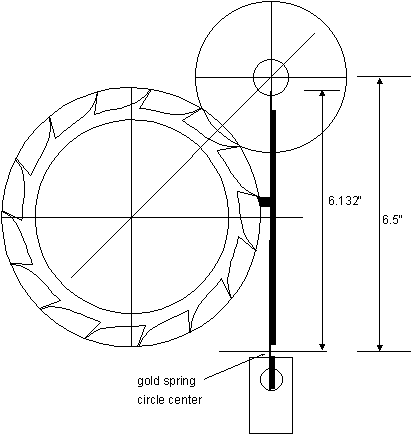Rotate the detent arm and gold spring assembly clockwise by 1ş, until the detent pallet just releases the escape tooth. Rotate the discharge pallet counterclockwise by 8ş. Draw the impulse pallet at the edge of the impulse pallet's circle, just inside the escape wheel's circle.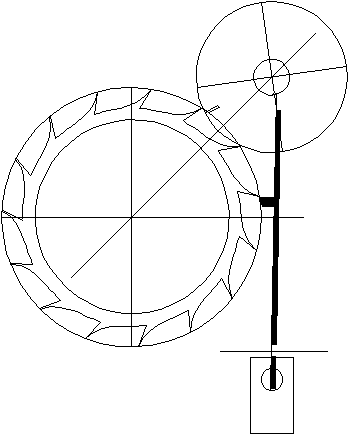Rotate the detent clockwise by 1ş extra, and the discharge and impulse pallets counterclockwise until the discharge pallet meets the gold spring. Then rotate the escape wheel until it meets the impulse pallet. The discharge and impulse pallets must rotate by enough to allow the escape wheel to move forwards until the tooth clears the detent before the detent is released. Otherwise, there will be some bent escape teeth.

Go to Chapter 21